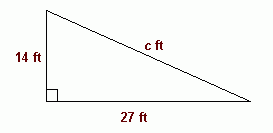SEARCH HOMEMath Central Quandaries & QueriesQuestion from Colburn: 27 feet long base 14 feet height what is the diagnalHi Colburn.Here's a picture of your question.

The answer is in the subject line you typed in:

a2 + b2 = c2

We use this formula with right triangles to find the length of an unknown side when the lengths of the other two sides are known. The longest side is the hypotenuse, and it is always opposite the right angle (90 degree angle) of the triangle. We always label its length c and the other two "legs" are a and b (it doesn't really matter which is which).

So in your question, you want to know c, and a and b are 27 and 14.
Can you calculate what c is if a2 + b2 = c2 ?

Stephen La Rocque.Math Central is supported by the University of Regina and The Pacific Institute for the Mathematical Sciences.